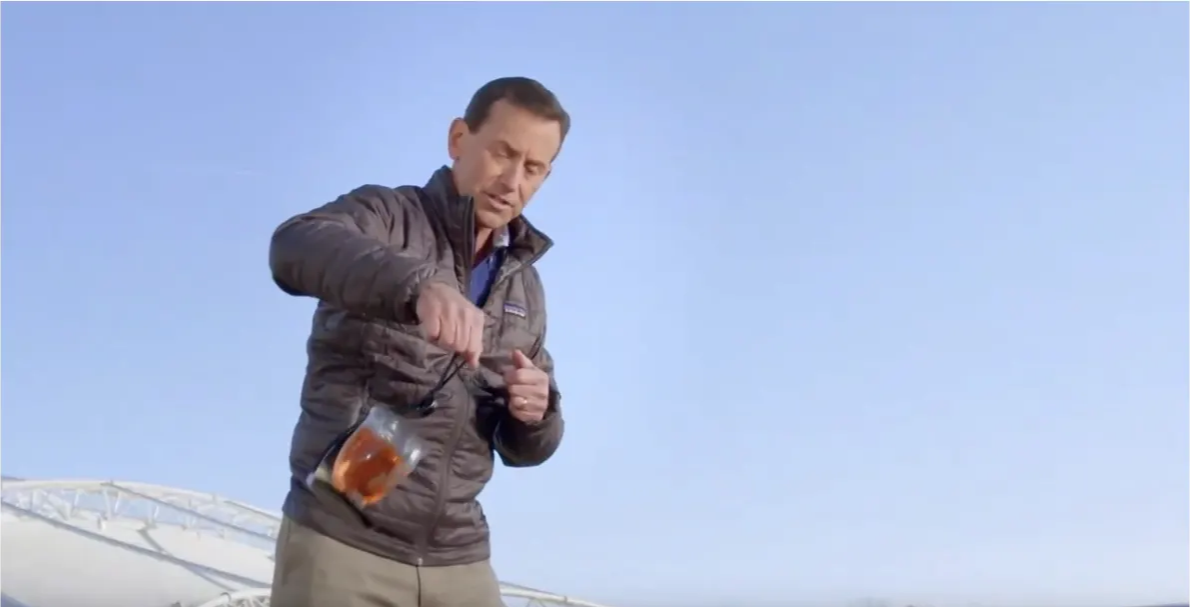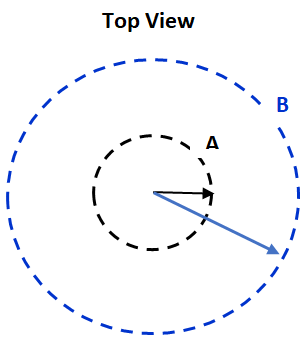# Centripetal Force Demonstrated With a Twirling Tray

The swinging bucket of water has been a teacher favorite for centuries. Teachers typically swing the bucket in a horizontal circle and tell students that the reason for the water not spilling is “centripetal force.” Some of the teachers might swing the bucket of water in a vertical circle and use the same argument that the “centripetal force” keeps the water in the bucket. This poor explanation gives the students the wrong impression that “centripetal force” is an actual force like gravity, friction, etc.

This video, with proper instruction, will present a much deeper understanding of the forces acting on the tray, the glass, and the water.

Place the SpillNot® device on your desk so that students will notice it when they enter the classroom. Hopefully a student will ask, “What is it?” Now you have the perfect opportunity to show the video. Start the video and then pause right away when the presenter mentions the “Laws of Motion.” Ask students to review Newton’ First Law (Objects in motion tend to travel in a straight line at a steady speed) and Newton’s 2nd Law (Objects with unbalanced forces (Fnet) will always accelerate in the direction of the unbalanced force (Fnet)). The equation is:

Now re-start the video to show the pitcher swinging in a circle. Pause where indicated and ask students to identify the forces acting on the SpillNot device (The forces are the pull of gravity and also the pull from the string and a small friction force.)

Sketch the demo and also draw and label the directions of the forces. (See below.)

Ask students to identify the forces on the pitcher. (The forces are the pull of gravity and also the push from the SpillNot device.)Ask students to compare the SpillNot® hanger to the waiter’s tray. How are they similar? (Both will push the glass and water to create a curved path.)

Now show the remainder of the video. Ask your students for their questions.

If you have a background in automotive engineering you might be able to explain how “electronic stability control” works. Otherwise use the “angle of lean” and the banking of the curves to make a smoother connection with the video.

Note – The video was shot outside to prevent collisions with the swinging tray.

When you complete the activities below, use a plastic wine glass to contain the water and be careful that the SpillNot® does not hit the ceiling or walls. (Is the ceiling higher in the hallway?)

I often do the demos first and then invite a student to try it to prove that physics is NOT MAGIC!

Also, be sure to emphasize that the centripetal force is not a separate force! The term “centripetal force” is used to indicate that the unbalanced force (Fnet) is toward the center of a curve. Remind students that the acceleration must always be in the same direction as the Fnet (toward the center of the curve.)

# Circular Motion Labs

If your Students (or you) have some difficulty in understanding circular motion. Here are a few tips and activities to correct their preconceptions.

Pre quiz:

Ask students to make a drawing of the moon orbiting the sun. Use a dotted line to show the shape of the orbit. Now ask them the draw an arrow on the moon to indicate the direction of motion. Next ask the students to list the force(s) acting on the moon to keep it in orbit. Now draw arrow next to the moon to indicate the directions of the forces. Are the forces balanced or unbalanced? Explain.

Have students share their ideas with their neighbors. Typical drawing is show here:

Demo #1: Tennis Ball on a String

We will swing a tennis ball in a circular orbit to “model” the motion of the moon around the earth.

Ask the students to name the horizontal forces acting on the ball. (Friction is small enough to neglect.)

Typical responses are: string and an outward (centrifugal) force.

Ask another student to be a catcher. Now again swing the ball and release the string exactly when the string is pointing to the catcher. What happens? (The ball goes in a direction tangent to the circle, NOT toward the catcher!) Conduct a robust discussion that concludes that there is NO outward force. Now write the word centrifugal and emphasize that the “f” in centrifugal is for fiction =  NOT real!

Key concept: Now ask student to revise their drawings of the earth and moon. There is NO outward force.   The only force on the diagram should be the gravity pull from the earth. Therefore, the forces are unbalanced (toward the earth.) A force that causes a circular motion is named a centripetal force.

See the “Curve Ball Demo” and then use the Arbor Scientific kit # P6-7900 so that your own students can better understand the forces needed for circular motion.

Unbalanced forces (called Fnet) always cause accelerations in the direction of the unbalanced force so add an arrow to the drawing to indicate the direction of the acceleration. (See the red arrow on the original drawing.)

Key concept

Objects moving in a circle always require an unbalanced force (Fnet) toward the center and an acceleration toward the center. Ask students to add the velocity, Fnet, and acceleration arrows for the positions here:

Now have students compare their completed drawings to the each other and then post the KEY.

The centripetal acceleration can be calculated as follows:

Spill NOT® Demo

Take a plastic glass and pour it ¾ full with a color liquid. (Add a few drops of food coloring to water.) Ask students to observe the angle of the top of the liquid. (The top of the liquid is parallel to the floor.) Now ask to again observe as you slowly walk in a straight line at a steady speed. (Same results!)

What happens to the platform and the water when I am moving forward and the stop? (The platform swings forward and the water stays parallel to the platform.)

Watch as I walk in a rectangular path (4 of 90 degree turns). (The Spill NOT swings out on the turns and the top of the water stays parallel to the platform!) What is the pattern for the motion of the platform and water? (The platform always “leans” in the direction of the acceleration.)

Extension

Use a sturdy length of 1 meter rope to hang the platform. Ask students to predict what will happen to the water when the platform is swung like the tennis ball in the previous demo. Ask students to “check your neighbor” for their prediction. Then call on a student to tell the class what her neighbor said. Then ask the class if anyone has a different opinion.

Next, have a student hold the end of the rope and slowly swing the platform in a circular motion. Ask the student to increase the speed until platform is moving fast. Ask students to observe the level of the water in the glass. (The water always stays parallel to the platform!)

Now share a sketch of the string, the platform, the glass, and the water.

What are the forces acting on the platform, glass, and water “system”? (Neglect air friction.)

Draw and label the forces. (In RED above.)

Are the forces balanced or unbalanced? How do you know?

Draw the force diagram on the right. The rope force pulls up enough to balance the downward pull from gravity. The sideways part of the rope force is the unbalanced or net force! Also, the rope and gravity forces are NOT balanced, because they are not equal and opposite. Also, the system is moving in a circle, so the system is accelerating toward the center.

The inward acceleration is caused by the Fnet towards the center! This Fnet called a centripetal force. Ask students to draw and label the Fnet and the “a” arrow on the drawing. (See blue arrows.)

More student demos

This activity requires two students to walk in circles. Student A’s circle has a radius is 1.0 meter. Student B’s circle has a radius of 2.0 meters. See sketch.

Ask student B to stay even with student A so that they take the same time to complete each circle.

Ask the rest of the class to watch closely and to notice that they are leaning inward. The students will definitely lean into the center.

Ask, "Are the students accelerating?” (Yes!) How do you know? (They are moving in circles.) What is the force that is pushing the students toward the center? (The friction from the floor is the Fnet or the floor provides the centripetal force.)Now the angle of lean depends on the value of the acceleration:

Student B has more acceleration as indicated by a larger angle of lean.

For example, if the angle of lean is 45 degrees, then

a = (10) tan (45o) =   (10) (1.0) = 10 m/s/s

The same equation can be used for the swinging tennis ball or the glass on the swinging platform.

When the speed of the swinging ball is faster, then the angle will be greater.

If the angle is 60 degrees, then the acceleration is:

a = g tan Q = (10) tan(60) = (10) (1.73) = 17.3 m/s/s

Application to safe driving

When a vehicle travels in a curve on a level road, the friction with the road pushes the car toward the center. When weather conditions, such as water or ice are on the road, the friction will be less, and the vehicles tend to travel off the road, in a straight line at a steady speed.

One way to overcome this problem is to design inclines into the curves. Have you ever seen the racetracks for cars? The curves are banked at large angles. This banking of the curves pushes the cars toward the center of the curve and reduces the need for friction!

If the banking angle matches the car speed and the radius of the curve, then the road supplies all of the necessary inward force. (NO friction is needed!)

Suppose that the car is moving at 90 m/s and the radius of the curve is 400 meters.

For a quick check, ask students to use the internet to find the banking angle in the curves at several racetracks such as Bristol, TN or Daytona, FL etc.

Explore the science of car crashes at classroom.iihs.orgMark Davids